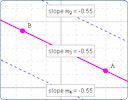Search IntMath
Close

450+ Math Lessons written by Math Professors and Teachers

5 Million+ Students Helped Each Year

1200+ Articles Written by Math Educators and Enthusiasts

Simplifying and Teaching Math for Over 23 Years

# IntMath Newsletter: New interactive graphs, ethical math help, resources

By Murray Bourne, 30 Nov 2011

30 Nov 2011

1. Explore basic graph concepts and understand them better
2. My dilemma - ethical math help
3. Resources
5. Friday math movies
6. Final thought - mistakes and learning

## 1. Explore basic graph concepts and understand them betterHere are some interactive graphs that help you better understand distance, slope, parallel lines and perpendicular lines. The basic concepts in plane geometry (like distance and slope) are vital for understanding later math topics (like calculus).

I recently added new pages in the Plane Analytic Geometry chapter in IntMath. Each one now has an interactive graph that helps you to understand how the formula works.

Check them out:

Distance Formula

Gradient of a Line, and Inclination

Parallel Lines

Perpendicular Lines

I'm hoping the interactive JSXGraphs are useful for both students and teachers. Please tell me what you think of them. Any improvements you can suggest which would make them more useful for you?

[Please give your suggestions in the comments section at the end of this post: Explore basic graph concepts and understand them better]

## 2. My dilemma - ethical math help

Is there a difference between paying someone to do your math homework and getting a computer to do it? Are they both unethical?

I'd be interested in your thoughts on this. See:

## 3. Resources

Manga High is a "new games-based maths teaching resource". For example, Pyramid Panic requires you to "use your geometry skills to build a path across the voids, and escape from the pyramid, staying ahead of the mongrel demon Ammit. Battle skull bats and skeleton thieves with your ankh cross." Topics covered in this game include Tigonometry, Pythagoras, Areas, Perimeters and Circumference. There are free "lite" versions, and paid versions allow social interaction with students from other schools.

GeoGebra examples: Analemma shares some interactive GeoGebra applets that illustrate mathematical concepts. Some of the topics include Bayes' Theorem, Circle as a limit of polygons, Circles of Apollonius, Domain and Range, Ellipse in a Box, and Eratosthenes measures Earth. Being java-based applets, they take a long time to load, but it's worth the wait.

Here's an interesting idea. Twylah gives you a summary of Twitter tweets in a newspaper style. Here are recent IntMath tweets in this form:

IntMath Tweets on Twylah

## 5. Friday math movies

Suitable for: Everyone, but especially teachers.(a) Math comes to life Here is an excellent way to animate math concepts. Friday math movie: Math comes to life

Suitable for: Everyone, especially if you are on Facebook (and who isn't?).(b) Facebook for Math Nerds This is first in a series of funny videos for math nerds. You'll enjoy it even if you are normal! Friday math movie: Facebook for Math Nerds

## 6. Final thought - mistakes and learning

This is from author John Powell, who could have been talking about learning math!

"The only real mistake is the one from which we learn nothing." [John Powell]

Until next time, enjoy whatever you learn.

### 2 Comments on “IntMath Newsletter: New interactive graphs, ethical math help, resources”

Thank you sir for this excellent and informative articles.

2. Isidro Canales Barbosa says:

Thanks for the information. Its great website of math.

Isidro Canales Barbosa
Mexico

### Comment Preview

HTML: You can use simple tags like <b>, <a href="...">, etc.

To enter math, you can can either:

1. Use simple calculator-like input in the following format (surround your math in backticks, or qq on tablet or phone):
a^2 = sqrt(b^2 + c^2)
(See more on ASCIIMath syntax); or
2. Use simple LaTeX in the following format. Surround your math with $$ and $$.
$$\int g dx = \sqrt{\frac{a}{b}}$$
(This is standard simple LaTeX.)

NOTE: You can mix both types of math entry in your comment.